# How many

How many integers are greater than 547/3 and less than 931/4?

n =  50

### Step-by-step explanation: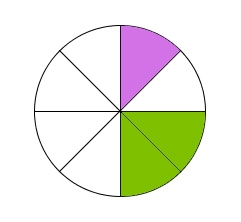Did you find an error or inaccuracy? Feel free to write us. Thank you!Tips to related online calculators
Need help to calculate sum, simplify or multiply fractions? Try our fraction calculator.
Do you want to round the number?
Do you want to perform natural numbers division - find the quotient and remainder?

## Related math problems and questions:

• RemaindersIt is given a set of numbers { 170; 244; 299; 333; 351; 391; 423; 644 }. Divide this numbers by number 66 and determine set of remainders. As result write sum of this remainders.
• Fractions and mixed numerals(a) Convert the following mixed numbers to improper fractions. i. 3 5/8 ii. 7 7/6 (b) Convert the following improper fraction to mixed number. i. 13/4 ii. 78/5 (c) Simplify these fractions to their lowest terms. i. 36/42 ii. 27/45 2. evaluate following ex
• Area 4gonCalculate the area of 4-gon, two, and the two sides are equal and parallel with lengths 11, 5, 11, and 5. Inner angles are 45°, 135°,45°, 135°.
• Pupils 7There are 40 pupils in a certain class. 3/5 of the class are boys. How many are girls?
• Evaluate mixed expressionsWhich of the following is equal to 4 and 2 over 3 divided by 3 and 1 over 2? A. 4 and 2 over 3 times 3 and 2 over 1 B. 14 over 3 times 2 over 7 C. 14 over 3 times 7 over 2 D. 42 over 3 times 2 over 31
• Hotel 4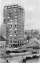A 360 room hotel has 1/3 of its rooms occupied at present . How many rooms are empty?
• CompareCompare fractions (34)/(3) and (12)/(4). Which fraction of the lower?
• Divisible by fourAre 240 divisible by 4?
• Reminder and quotientThere are given numbers A = 135, B = 315. Find the smallest natural number R greater than 1 so that the proportions R:A, R:B are with the remainder 1.
• Plums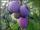In the bag was to total 136 plums. Igor took 3 plums and Mary took 4/7 from the rest. How many plums remained in the bag?
• Yogurt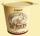The board is 35 yogurts. How many boards of yogurts must be ordered for 886 people in the canteen?
• ShirtsIn a classroom, 1/6 of the students are wearing blue shirts and 2/3 are wearing white shirts. There are 36 students in the class. How many students are wearing a shirt other than blue or white?
• Candies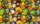There are 150 candies in the bowl. Ivan took 7 thirtieths, Charles 6 fifteenths, and Thomas 3 tenths. How many sweets are left in the bowl?
• PointsGryffindor won 437 points. How many points obtained by each of the faculties if they were split at a ratio of 5: 7: 3: 4?
• How many 14How many 1/2 cup serving are in a package of cheese that contains 5 1/4 cups altogether?
• Potatoes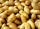Could 446 tons of potatoes (ρ = 771 kg/m³) fit in a warehouse with a volume of 699 m³?
• AthletesAthletes at the stadium could enter two-steps, three-steps, four-steps, five-steps, six-steps. There were more than 100 but less than 200. How many athletes were there?